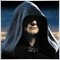# 4002: ERR_ARRAY_INDEX_OUT_OF_RANGE[Deleted]

Hi

I'd like to show you a snippet which constantly gives me the 4002 error. Which means, if I comment this function out (obviously without the Print() function ;) ), the error disappears. What the code does: it calculates the average of the trueranges of the last 20 days.

```double truerange;
double Diff_HiLo;
double Diff_HiPrevDay;
double Diff_LoPrevDay;
double n;

void calculateN()
{

i = 1;
while(i <= 20)
{
Diff_HiLo[i-1] = iHigh(NULL,PERIOD_D1,i-1) - iLow(NULL,PERIOD_D1,i-1);
Diff_HiPrevDay[i-1] = iHigh(NULL,PERIOD_D1,i-1) - iClose(NULL,PERIOD_D1,i);
Diff_LoPrevDay[i-1] = iClose(NULL,PERIOD_D1,i) - iLow(NULL,PERIOD_D1,i-1);

if (Diff_HiLo[i-1] > Diff_HiPrevDay[i-1])
{
truerange[i-1] = Diff_HiLo[i-1];
}else
{
truerange[i-1] = Diff_HiPrevDay[i-1];
}

if (Diff_LoPrevDay[i-1] > truerange[i-1])
{
truerange[i-1] = Diff_LoPrevDay[i-1];
}
i++;
}

n = 0;
i = 1;
while(i <= 20)
{
n=n+truerange[i-1];
i++;
}
n=n/20;

Print(GetLastError());

}```

Thank you!1091

Well I can't see any obvious error. Why don't you comment half the function out and see if the error goes away, and keep halving the number of lines commented out until you find the line or lines which cause the problem?

Additionally you are doing a heck of a lot of i-1 calculations. It would be more efficient to use i and on the occasion when you need it use an i+1.[Deleted]

Thank you for your answer!

Yes, this with the i is some habit of me :D

I forgot to mention that: No matter if I comment the first or the second loop out, the error occurs. Only if it's the whole function it stops to occur.[Deleted]

Another test: If I comment out the first loop, and take the truerange[i] out of the second loop (so there is no array left) I still get the error! There doesnt even exist any other array in the whole EA!

Ok, I took the snippet out of the code and put it in a new EA: no more errors.

I checked on the page for runtime errors: Can occur with ObjectName(), StringGetChar and StringSetChar. Well, I never used one of them.1091

I put your snippet into a script. No error. (After I defined i )

```double truerange;
double Diff_HiLo;
double Diff_HiPrevDay;
double Diff_LoPrevDay;
double n;

int i;

void start()
{

i = 1;
while(i <= 20)
{
Diff_HiLo[i-1] = iHigh(NULL,PERIOD_D1,i-1) - iLow(NULL,PERIOD_D1,i-1);
Diff_HiPrevDay[i-1] = iHigh(NULL,PERIOD_D1,i-1) - iClose(NULL,PERIOD_D1,i);
Diff_LoPrevDay[i-1] = iClose(NULL,PERIOD_D1,i) - iLow(NULL,PERIOD_D1,i-1);

if (Diff_HiLo[i-1] > Diff_HiPrevDay[i-1])
{
truerange[i-1] = Diff_HiLo[i-1];
}else
{
truerange[i-1] = Diff_HiPrevDay[i-1];
}

if (Diff_LoPrevDay[i-1] > truerange[i-1])
{
truerange[i-1] = Diff_LoPrevDay[i-1];
}
i++;
}

n = 0;
i = 1;
while(i <= 20)
{
n=n+truerange[i-1];
i++;
}
n=n/20;

Print(GetLastError());

}```[Deleted]

Yeah I forgot to copy the i definer.

Let's sum up:

The error only occurs in context of the whole script

If I run the EA without this function, the error is gone

It is not possible to detect the error in the script (if only something of the function runs, there's the error)

there aren't any other arrays in the EA

I only refer to "n" in the rest of the EA (and the calculated value could be correct, I'm not 100% sure)

The function is called in the start() function

Is there a possibility to clear the GetLastError? I could see how many times the error really occurs17374

Sasmo:

Is there a possibility to clear the GetLastError? I could see how many times the error really occurs

It is cleared when you call it.

Are you compiling in a different Build compared to the Build you are running your code in ? what Builds are your Editor and MT4 Terminal ?1091

Sasmo:

The error only occurs in context of the whole script

If I run the EA without this function, the error is gone

It is not possible to detect the error in the script (if only something of the function runs, there's the error)

there aren't any other arrays in the EA

I only refer to "n" in the rest of the EA (and the calculated value could be correct, I'm not 100% sure)

The function is called in the start() function

So something in this function is changing something used in the rest of the program?

Are you using i to index anything when you return from the function for example?